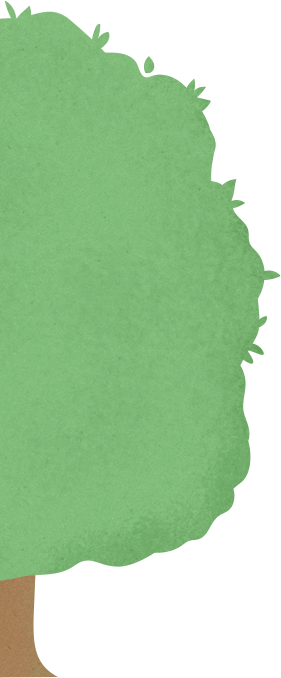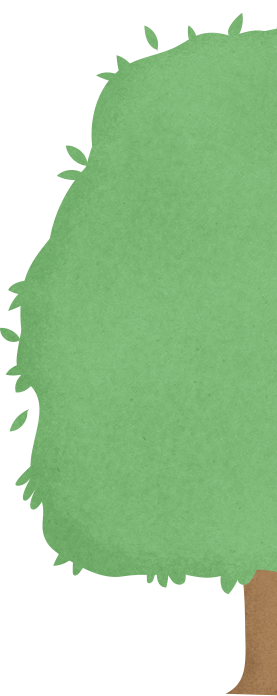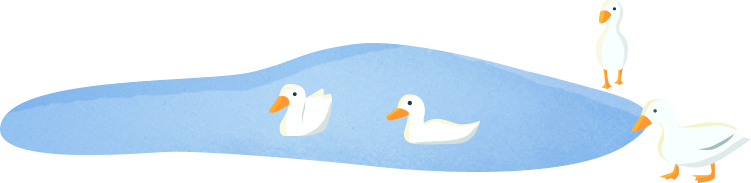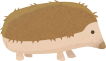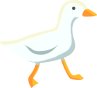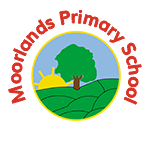## Maths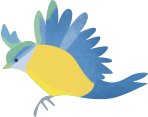# Maths

Please use the maths hub resources/sheets this week. These can be found in the Year 1 section of the school website.

Underneath for each day will be other activities that can be done.

Day 1

Play a game where you choose some counters/toys. Start off with an amount under ten and then add on another amount under ten

e.g 7 + 5 = 12   discuss counting on as a way to work out the answer, so counting the 7 and then counting on from that 5 more, not going back to the beginning and counting them all again from 1. You could make your questions into word problems such as: 6 people got on the bus (get 6 toys) then 8 more people got on (count 8 more toys). Demonstrate counting on from 6, to 8 more than 6.

Maths hub sheet day 1

Day 2

Find and make number bonds

How many different ways can you make 10? Then try, how many different ways can you make 20? E.g 22 – 2 = 20   13 + 7 = 20

Use this video to help: https://www.youtube.com/watch?v=h6udqW6VhWg

Maths hub sheet day 2

Day 3

Practise making addition easier by trying to get to ten first e.g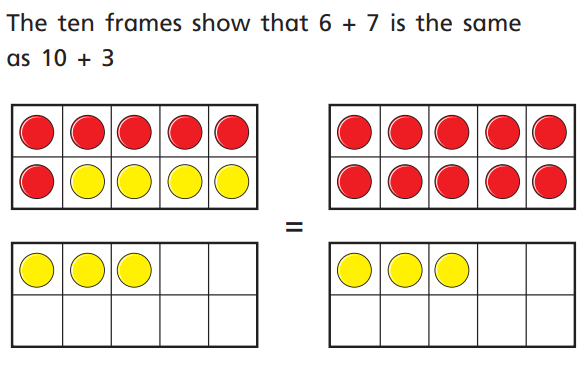Can you work out these as well using the same technique?

5 + 6 =       or     10 + __ =

8 + 4 =       or     10 + __ =

7 + 8 =       or     10 + __ =

Maths hub sheet day 3

Day 4

Subtraction not crossing 10

Draw circles to represent numbers and then cross out to show taking away. You can also count out the whole amount and then physically take away some.

Work out these calculations:

12 – 4 =

15 – 6 =

18 – 9 =

20 – 1 =

Maths hub sheet day 4

Day 5

Subtraction crossing 10

Using a number line work out these calculations: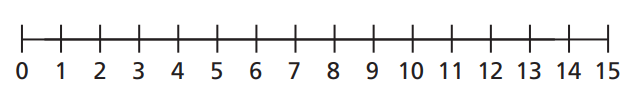Like this: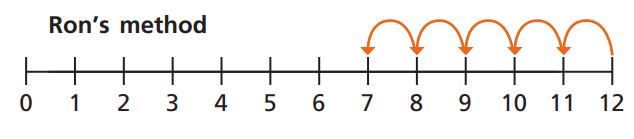15 – 3 =

11 – 5 =

14 – 8 =

13 – 9 =

Maths hub sheet day 5